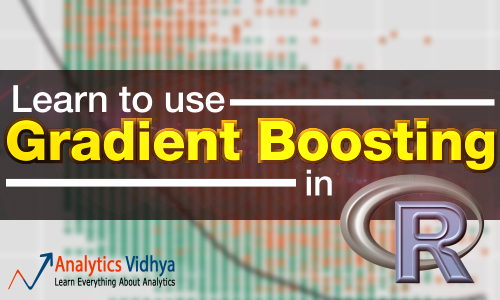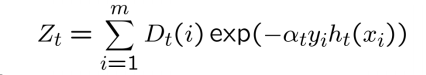Home » Learn Gradient Boosting Algorithm for better predictions (with codes in R)

# Learn Gradient Boosting Algorithm for better predictions (with codes in R)

## Introduction

The accuracy of a predictive model can be boosted in two ways: Either by embracing feature engineering or by applying boosting algorithms straight away. Having participated in lots of data science competition, I’ve noticed that people prefer to work with boosting algorithms as it takes less time and produces similar results.

There are multiple boosting algorithms like Gradient Boosting, XGBoost, AdaBoost, Gentle Boost etc. Every algorithm has its own underlying mathematics and a slight variation is observed while applying them. If you are new to this, Great! You shall be learning all these concepts in a week’s time from now.

In this article, I’ve explained the underlying concepts and complexities of Gradient Boosting Algorithm. In addition, I’ve also shared an example to learn its implementation in R.

Note: This guide is meant for beginners. Hence, if you’ve already mastered this concept, you may skip this article here.## Quick Explanation

While working with boosting algorithms, you’ll soon come across two frequently occurring buzzwords: Bagging and Boosting. So, how are they different? Here’s a one line explanation:

Bagging: It is an approach where you take random samples of data, build learning algorithms and take simple means to find bagging probabilities.

Boosting: Boosting is similar, however the selection of sample is made more intelligently. We subsequently give more and more weight to hard to classify observations.

Okay! I understand you’ve questions sprouting up like ‘what do you mean by hard? How do I know how much additional weight am I supposed to give to a mis-classified observation.’ I shall answer all your questions in subsequent sections. Keep Calm and proceed.

## Let’s begin with an easy example

Assume, you are given a previous model M to improve on. Currently you observe that the model has an accuracy of 80% (any metric). How do you go further about it?

One simple way is to build an entirely different model using new set of input variables and trying better ensemble learners. On the contrary, I have a much simpler way to suggest. It goes like this:

`Y = M(x) + error`

What if I am able to see that error is not a white noise but have same correlation with outcome(Y) value. What if we can develop a model on this error term? Like,

`error = G(x) + error2`

Probably, you’ll see error rate will improve to a higher number, say 84%. Let’s take another step and regress against error2.

`error2 = H(x) + error3`

Now we combine all these together :

`Y = M(x) + G(x) + H(x) + error3`

This probably will have a accuracy of even more than 84%. What if I can find an optimal weights for each of the three learners,

`Y = alpha * M(x) + beta * G(x) + gamma * H(x) + error4`

If we found good weights, we probably have made even a better model. This is the underlying principle of a boosting learner. When I read the theory for the first time, I had two quick questions:

1. Do we really see non white noise error in regression/classification equations? If not, how can we even use this algorithm?
2. Wow, if this is possible, why not get near 100% accuracy?

I’ll answer these questions in this article, however, in a crisp manner. Boosting is generally done on weak learners, which do not have a capacity to leave behind white noise.  Secondly, boosting can lead to overfitting, so we need to stop at the right point.

## Let’s try to visualize one Classification Problem

Look at the below diagram :We start with the first box. We see one vertical line which becomes our first week learner. Now in total we have 3/10 mis-classified observations. We now start giving higher weights to 3 plus mis-classified observations. Now, it becomes very important to classify them right. Hence, the vertical line towards right edge. We repeat this process and then combine each of the learner in appropriate weights.

### Explaining underlying mathematics

How do we assign weight to observations?

We always start with a uniform distribution assumption. Lets call it as D1 which is 1/n for all n observations.

Step 1 . We assume an alpha(t)

Step 2: Get a weak classifier h(t)

Step 3: Update the population distribution for the next stepStep 4 : Use the new population distribution to again find the next learner

Scared of Step 3 mathematics? Let me break it down for you. Simply look at the argument in exponent. Alpha is kind of learning rate, y is the actual response ( + 1 or -1) and h(x) will be the class predicted by learner. Essentially, if learner is going wrong, the exponent becomes 1*alpha and else -1*alpha. Essentially, the weight will probably increase if the prediction went wrong the last time. So, what’s next?

Step 5 : Iterate step 1 – step 4 until no hypothesis is found which can improve further.

Step 6 : Take a weighted average of the frontier using all the learners used till now. But what are the weights? Weights are simply the alpha values. Alpha is calculated as follows:

## Time to Practice – Example

I recently participated in an online hackathon organized by Analytics Vidhya. For making the variable transformation easier, I combined both test and train data in the file complete_data. I started with basic import function and splitted the population in Devlopment, ITV and Scoring.

```library(caret)
rm(list=ls())
setwd("C:\\Users\\ts93856\\Desktop\\AV")
library(Metrics)```
```complete <- read.csv("complete_data.csv", stringsAsFactors = TRUE)
train <- complete[complete\$Train == 1,]
score <- complete[complete\$Train != 1,]```
`set.seed(999)`
```ind <- sample(2, nrow(train), replace=T, prob=c(0.60,0.40))
trainData<-train[ind==1,]
testData <- train[ind==2,]```
```set.seed(999)
ind1 <- sample(2, nrow(testData), replace=T, prob=c(0.50,0.50))
trainData_ens1<-testData[ind1==1,]
testData_ens1 <- testData[ind1==2,]```
```table(testData_ens1\$Disbursed)/nrow(testData_ens1)
#Response Rate of 9.052%```

Here is all you need to do, to build a GBM model.

`fitControl <- trainControl(method = "repeatedcv", number = 4, repeats = 4)`
```trainData\$outcome1 <- ifelse(trainData\$Disbursed == 1, "Yes","No")
set.seed(33)
gbmFit1 <- train(as.factor(outcome1) ~ ., data = trainData[,-26], method = "gbm", trControl = fitControl,verbose = FALSE)```
```gbm_dev <- predict(gbmFit1, trainData,type= "prob")[,2]
gbm_ITV1 <- predict(gbmFit1, trainData_ens1,type= "prob")[,2]
gbm_ITV2 <- predict(gbmFit1, testData_ens1,type= "prob")[,2]```
```auc(trainData\$Disbursed,gbm_dev)
auc(trainData_ens1\$Disbursed,gbm_ITV1)
auc(testData_ens1\$Disbursed,gbm_ITV2)

```

As you will see after running this code, all AUC will come extremely close to 0.84 . I will leave the feature engineering upto you, as the competition is still on. You are welcome to use this code to compete though. GBM is the most widely used algorithm. XGBoost is another faster version of boosting learner which I will cover in any future articles.

## End Notes

I have seen boosting learners extremely quick and highly efficient. They have never disappointed me to get high initial scores on Kaggle and other platforms. However, it all boils down to how well can you do feature engineering.

Have you used Gradient Boosting before? How did the model perform? Have you used boosting learners in any other capacity. If yes, I would love to hear your experiences in the comments section below.

### If you like what you just read & want to continue your analytics learning, subscribe to our emails, follow us on twitter or like our facebook page

You can also read this article on our Mobile APP•Vishwajyoti says:

Nicely explained . I have used ada boosting and the performance of random forest and ada boosting were almost same in my case. However, I was wondering if different types of boosting have any advantage over one another.

•Krishna Priya says:

Thank you Tavish for the wonderful post. I have used Ada Boost Algorithm. I was just comparing the performance of Ada Boost , Random Forest , SVM , and Decision Tree Induction with various data sets and various metrics. Ada just dominated than all others and showed an accuracy of approximately 98-99% with all data sets .

•venugopal says:

Yes this is the Essenble methods ERA…

•Krishna Priya says:

Thank you for the wonderful post. I worked with Ada Boost Algorithm quite a while back. I was just comparing the performance of Ada Boost, SVM, Random Forest and Decision Tree with various data sets and metrics . Ada Boost performed far better than others and showed an accuracy of 98-99 % in all cases .

•vishwa says:

Hi,
while running the below code , i am getting the error “Error: cannot allocate vector of size 56.4 Gb”
code:
gbmFit1 <- train(as.factor(outcome1) ~ ., data = trainData[,-26], method = "gbm", trControl = fitControl,verbose = FALSE)
I tried to fix this issue with "memory.limit(size=)" and gc() , but of no use.I am running on 64bit machine.

•Kunal Jain says:

Vishwa,

If you have not posted it already, you should post this on our discussion portal.

Regards,
Kunal

•Tavish says:

Are you using some kind of ID in the independent variables. Just check, else this should not come as an error for this dataset.

•Arindam says:

Tavish

What do you mean by ids in predictors. Were you implying that if a predictor has high amount of categories or cluster this could happen. I also keep getting this error not for this code but on my other projects I am working on

•Ram says:

Vishwa,

Were you able to solve the problem?
If the data size is beyond your machine’s capacity, you can try fitting the model on a random subset of the data.

numrows=nrow(trainData)
ind=sample(1:numrows, numrows/10) ## select only 10% of the data
trainData_s=trainData[ind,]

Then fit a model on trainData_s and see if the machine can handle this data.

•Kuber Jain says:

Hi,
How can I include weight variable as a part of above gradient boosting R code and get the parameter estimates of predictor variables?

•Pankaj says:

hi,

wonderful article; can you please share the data you used along the exercise above.

Many Thanks,

•Sharon says:

Thank you Tavish. This is a great article.
Quick question – epsilon refers to the error rate, right? (in step 6). That would indicate higher weights to trees with lower error rate. Just want to confirm.

Also, to work with larger amounts of data, you can adjust your train fraction and bag fraction to choose a smaller set of rows for each tree, but set a higher number of trees so it converges well.

Also, # variables makes a huge difference in memory requirements. If you have a few hundred variables to work with, you may want to split into multiple groups, build separate GBM models and then combine the best variables from each iteration.

One thing the examples may be missing is taking care of overfitting. Adjusting final node size is important.

•Yasho Joshi says:

Can you please attach a link to the dataset that used to explain this method?

•Rich says:

Echoing previous requests: can you please post the data set???

•Krishna says:

Is this really Gradient Boosting? I don’t see any loss function in this post, It just seems to be AdaBoost. Could you please clarify?